• 1、一维离散傅里叶变换DFT。 DFT:(Discrete Fourier Transform)离散傅里叶变换是傅里叶变换在时域和频域上都呈离散的形式，将信号的时域采样变换为其DTFT的频域采样。在形式上，变换两端（时域和频域上）的序列是...
一、介绍

1、一维离散傅里叶变换DFT。

DFT:(Discrete Fourier Transform)离散傅里叶变换是傅里叶变换在时域和频域上都呈离散的形式，将信号的时域采样变换为其DTFT的频域采样。在形式上，变换两端（时域和频域上）的序列是有限长的，而实际上这两组序列都应当被认为是离散周期信号的主值序列。即使对有限长的离散信号作DFT，也应当将其看作其周期延拓的变换。

根据欧拉公式，其中，i为虚数单位，即。

公式：，可看作：。

2、一维逆离散傅里叶变换IDFT。

公式：，可看作：

3、一维快速傅里叶变换FFT。

1)FFT（Fast Fourier Transformation）是离散傅氏变换（DFT）的快速算法，即利用计算机计算离散傅里叶变换（DFT)的高效、快速计算方法的统称。快速傅里叶变换是1965年由J.W.库利和T.W.图基提出的。采用这种算法能使计算机计算离散傅里叶变换所需要的乘法次数大为减少，特别是被变换的抽样点数N越多，FFT算法计算量的节省就越显著。即为快速傅氏变换。它是根据离散傅氏变换的奇、偶、虚、实等特性，对离散傅立叶变换的算法进行改进获得的。

2)因为根据欧拉公式和一维离散傅里叶公式，可以将欧拉公式中的看作是，而且可以是要将其代入到cos和sin函数中，所以其实可以看作是一个半径为1的圆。而可以看作将圆分成N份，即上面那个矩阵中的，而第k份的那个点就是那个值。

3)可以推导出关系。

周期性：  ,

原点对称：

4）要让DFT的长度等于2的n次幂，其中n是正整数。

令  ，  ，

则。

但是这里的k是小于的，但是可以推导出

，  其中。

最后将G(x)，P(x)按照这个方式，用分治法不停的拆分出来，剩下最后俩个 ，然后返回计算的结果。

4、一维逆快速傅里叶变换IFFT。

比较DFT和IDFT公式，会发现逆变换和正变换的区别在于：

1、中的正负的区别。

2、逆变换在结束之后，每个值都要除以原数组的大小。

所以我们只要套用FFT的算法就可以了，将F(k)作为输入数组，输出f(x)作为输出数组，将因子改为,最后将f(x)全部除以N就可以了。

二、主要代码

1、复数类。

package com.zxj.reptile.utils.number;

public class Complex {
private double real;//实数
private double image;//虚数

public Complex() {
real = 0;
image = 0;
}

public Complex(double real, double image) {
this.real = real;
this.image = image;
}

//加：(a+bi)+(c+di)=(a+c)+(b+d)i
double real = complex.getReal();
double image = complex.getImage();
double newReal = this.real + real;
double newImage = this.image + image;
return new Complex(newReal, newImage);
}

//减：(a+bi)-(c+di)=(a-c)+(b-d)i
public Complex sub(Complex complex) {
double real = complex.getReal();
double image = complex.getImage();
double newReal = this.real - real;
double newImage = this.image - image;
return new Complex(newReal, newImage);
}

public Complex mul(Complex complex) {
double real = complex.getReal();
double image = complex.getImage();
double newReal = this.real * real - this.image * image;
double newImage = this.image * real + this.real * image;
return new Complex(newReal, newImage);
}

public Complex mul(double multiplier) {
return mul(new Complex(multiplier, 0));
}

public Complex div(Complex complex) {
double real = complex.getReal();
double image = complex.getImage();
double denominator = real * real + image * image;
double newReal = (this.real * real + this.image * image) / denominator;
double newImage = (this.image * real - this.real * image) / denominator;
return new Complex(newReal, newImage);
}

public double getReal() {
return real;
}

public void setReal(double real) {
this.real = real;
}

public double getImage() {
return image;
}

public void setImage(double image) {
this.image = image;
}

@Override
public String toString() {
String str = "";
if (real != 0) {
str += real;
} else {
str += "0";
}
if (image < 0) {
str += image + "i";
} else if (image > 0) {
str += "+" + image + "i";
}
return str;
}

//欧拉公式 e^(ix)=cosx+isinx
public static Complex euler(double x) {
double newReal = Math.cos(x);
double newImage = Math.sin(x);
return new Complex(newReal, newImage);
}

/**
* 比较两个复数是否相等，并可以调节小数精度误差
*
* @param a 复数a
* @param b 复数b
*/
public static boolean equals(Complex a, Complex b) {
return NumberUtils.getRound(a.getImage(), 4) == NumberUtils.getRound(b.getImage(), 4) &&
NumberUtils.getRound(a.getReal(), 4) == NumberUtils.getRound(b.getReal(), 4);
}

/**
* 比较两个复数数组是否相等，小数精度误差在6位数
*
* @param a 复数数组a
* @param b 复数数组b
*/
public static boolean equals(Complex[] a, Complex[] b) {
if (a.length != b.length) {
return false;
}
for (int i = 0; i < a.length; i++) {
if (!equals(a[i], b[i])) {
return false;
}
}
return true;
}

/**
* 将复数数组转换为double数组
*
* @param complexArray 复数数组
*/
public static double[] getDoubleArray(Complex[] complexArray) {
int length = complexArray.length;
double[] doubleArray = new double[length];
for (int i = 0; i < length; i++) {
doubleArray[i] = NumberUtils.getRound(complexArray[i].getReal(), 2);
}
return doubleArray;
}

/**
* 将double数组转换为复数数组
*
* @param doubleArray double数组
*/
public static Complex[] getComplexArray(double[] doubleArray) {
int length = doubleArray.length;
Complex[] complexArray = new Complex[length];
for (int i = 0; i < length; i++) {
complexArray[i] = new Complex(doubleArray[i], 0);
}
return complexArray;
}
}


2、工具方法。


/**
* value指定小数精度的四舍五入
*
* @param value 值
* @param decimalLength 精度值
*/
public static double getRound(double value, int decimalLength) {
double flag = Math.pow(10, decimalLength);
int a = (int) Math.round(value * flag);
return a / flag;
}

/**
* 随机生成一维数组
*
* @param length   数值长度
* @param minValue 最小值（包括）
* @param maxValue 最大值（包括）
*/
public static double[] getRandom(int length, int minValue, int maxValue) {
int range = maxValue - minValue + 1;
double[] array = new double[length];
for (int i = 0; i < length; i++) {
array[i] = (int) (Math.random() * range) + minValue;
}
return array;
}

3、一维离散傅里叶变换DFT和一维逆离散傅里叶变换IDFT。

/**
* 一维离散傅里叶变换DFT
*
* @param array 一维数组
*/
public static Complex[] getDft(double[] array) {
int N = array.length;
Complex[] complexArray = new Complex[N];
double flag = -2 * Math.PI / N;
for (int u = 0; u < N; u++) {
//x-N的累加
Complex sum = new Complex();
for (int x = 0; x < N; x++) {
//f(x) * e^(-(2 * PI * u * x / N)i)
Complex complex = Complex.euler(flag * u * x).mul(array[x]);
}
complexArray[u] = sum;
}
return complexArray;
}

/**
* 一维逆离散傅里叶变换IDFT
*
* @param complexArray 一维复数数组
*/
public static double[] getInverseDft(Complex[] complexArray) {
int N = complexArray.length;
double[] array = new double[N];
double flag = 2 * Math.PI / N;
for (int x = 0; x < N; x++) {
//u-N的累加
Complex sum = new Complex();
for (int u = 0; u < N; u++) {
//F(u) * e^((2 * PI * u * x / N)i)
Complex complex = Complex.euler(flag * u * x).mul(complexArray[u]);
}
//F(u) * e^((2 * PI * u * x / N)i) / N
sum = sum.mul(1.0 / N);
double realSum = sum.getReal();
array[x] = NumberUtils.getRound(realSum, 2);
}
return array;
}

4、一维快速傅里叶变换FFT和一维逆快速傅里叶变换IFFT，采用递归分治的方式。

    /**
* 一维快速傅里叶变换FFT
*
* @param array 一维数组
*/
public static Complex[] getFft(double[] array) {
//实际的长度
int length = array.length;
//调节过的长度
int variableLength = (int) NumberUtils.getVariablePow(length, 2);
Complex[] variableArray = new Complex[variableLength];
for (int i = 0; i < variableLength; i++) {
if (i < length) {
variableArray[i] = new Complex(array[i]);
} else {
variableArray[i] = new Complex();
}
}
return fftProgress(variableArray, -1);
}

/**
* 一维逆快速傅里叶变换IFFT  当N是2次幂时
*
* @param complexArray 一维复数数组
*/
public static double[] getInverseFft(Complex[] complexArray) {
return getInverseFft(complexArray, complexArray.length);
}

/**
* 一维逆快速傅里叶变换IFFT  当N不是2次幂时
*
* @param complexArray 一维复数数组
* @param realLength   返回的数组长度
*/
public static double[] getInverseFft(Complex[] complexArray, int realLength) {
int length = complexArray.length;
Complex[] resultArrays = fftProgress(complexArray, 1);//f(k)
double[] array = new double[realLength];
//每个数都要除以N
for (int i = 0; i < realLength; i++) {
array[i] = NumberUtils.getRound(resultArrays[i].getReal() / length, 2);
}
return array;
}

/**
* 一维快速傅里叶变换FFT和一维逆快速傅里叶变换IFFT递归过程
*
* @param complexArray 一维复数数组
* @param minus        FFT为1，IFFT为-1
*/
private static Complex[] fftProgress(Complex[] complexArray, int minus) {
int length = complexArray.length;
if (length == 2) {
//F(0)=f(0)+f(1),F(1)=f(0)-f(1)
return new Complex[]{
complexArray.sub(complexArray),};
} else if (length == 1) {
return complexArray;
}
int middle = length / 2;
//
Complex[] a = new Complex[middle];//a(x)=f(2x)
Complex[] b = new Complex[middle];//b(x)=f(2x+1)
for (int i = 0; i < middle; i++) {
a[i] = complexArray[2 * i];
b[i] = complexArray[2 * i + 1];
}
//
Complex[] complexesA = fftProgress(a, minus);//计算G(k)
Complex[] complexesB = fftProgress(b, minus);//计算P(k)
Complex[] resultArray = new Complex[length];//F(k)
double flag = minus * 2 * Math.PI / length;//2Pi*k/N
for (int i = 0; i < middle; i++) {
//e^(2Pi*k/N)
Complex complex = Complex.euler(flag * i).mul(complexesB[i]);
//F(k)=G(k)+(e^(2Pi*k/N))*P(k)
//F(k+(N/2))=G(k)+(e^(2Pi*(k+(N/2))/N))*P(k+(N/2))
resultArray[i + middle] = complexesA[i].sub(complex);
}
return resultArray;
}

三、FFT和DFT结果比较

1、主流程代码。

package com.zxj.reptile.test.image;

import com.zxj.reptile.utils.image.FourierUtils;
import com.zxj.reptile.utils.number.ArrayUtils;
import com.zxj.reptile.utils.number.Complex;

import java.util.Arrays;

public class FourierTest {
public static void main(String[] args) {
System.out.println("------开始------");
testDft(8);
System.out.println("------结束------");
}

private static void testDft(int length) {
System.out.println("原数据: ");
System.out.println(String.format("大小为%d", length));
double[] array = ArrayUtils.getRandom(length, 0, 255);
for (int i = 0; i < array.length; i++) {
System.out.println(array[i]);
}
System.out.println();
//
System.out.println("一维离散傅里叶变换DFT: ");
long time = System.currentTimeMillis();
Complex[] dftArray = FourierUtils.getDft(array);
System.out.println("花费时间 ：" + (System.currentTimeMillis() - time));
for (int i = 0; i < dftArray.length; i++) {
System.out.println(dftArray[i]);
}
System.out.println();
//
System.out.println("一维逆离散傅里叶变换IDFT: ");
time = System.currentTimeMillis();
double[] inverseDftArray = FourierUtils.getInverseDft(dftArray);
System.out.println("花费时间 ：" + (System.currentTimeMillis() - time));
for (int i = 0; i < inverseDftArray.length; i++) {
System.out.println(inverseDftArray[i]);
}
if (Arrays.equals(array, inverseDftArray)) {
System.out.println("IDFT成功");
} else {
System.out.println("IDFT失败");
}
System.out.println();
//
System.out.println("一维快速傅里叶变换FFT: ");
time = System.currentTimeMillis();
Complex[] fftArray = FourierUtils.getFft(array);
System.out.println("花费时间 ：" + (System.currentTimeMillis() - time));
for (int i = 0; i < fftArray.length; i++) {
System.out.println(fftArray[i]);
}
System.out.println();
//
System.out.println("一维逆快速傅里叶变换IFFT: ");
time = System.currentTimeMillis();
double[] inverseFFTArray = FourierUtils.getInverseFft(fftArray, array.length);
System.out.println("花费时间 ：" + (System.currentTimeMillis() - time));
for (int i = 0; i < inverseFFTArray.length; i++) {
System.out.println(inverseFFTArray[i]);
}
if (Arrays.equals(array, inverseFFTArray)) {
System.out.println("IFFT成功");
} else {
System.out.println("IFFT失败");
}
System.out.println();
}
}


2、IFFT和IFFT是否正确，当大小为8的时候，打印的日志如下。将FFT和DFT生成的数据进行对比，发现FFT变换成功。将IFFT生成的数据和原始的数据进行对比，发现IFFT变换成功。

------开始------
原数据:
大小为8
161.0
76.0
88.0
83.0
5.0
54.0
149.0
172.0

一维离散傅里叶变换DFT:
花费时间 ：16
788.0
234.48885271170673+108.37615433949871i
-71.00000000000007+124.99999999999993i
77.51114728829329-13.623845660501232i
18.0-8.682745805954733E-14i
77.51114728829299+13.623845660501132i
-71.00000000000018-125.0000000000002i
234.48885271170724-108.37615433949823i

一维逆离散傅里叶变换IDFT:
花费时间 ：0
161.0
76.0
88.0
83.0
5.0
54.0
149.0
172.0
IDFT成功

一维快速傅里叶变换FFT:
花费时间 ：0
788.0
234.48885271170678+108.3761543394987i
-71.00000000000001+125.0i
77.51114728829322-13.623845660501324i
18.0
77.51114728829323+13.623845660501303i
-70.99999999999999-125.0i
234.48885271170678-108.37615433949867i

一维逆快速傅里叶变换IFFT:
花费时间 ：0
161.0
76.0
88.0
83.0
5.0
54.0
149.0
172.0
IFFT成功

------结束------

2、当大小不为2次幂的时候，如6，FFT会在后面自动补0，知道2次幂为止，即8。但是这样子转换成频谱图会跟DFT的不一样，但是IFFT转换之后，再讲多余的给移除掉，最后的结果还是会跟原数据一样的。打印日志如下。

------开始------
原数据:
大小为6
82.0
41.0
232.0
90.0
183.0
11.0

一维离散傅里叶变换DFT:
花费时间 ：0
639.0
-189.50000000000003-68.41600689897068i
-61.49999999999996+16.45448267190423i
355.0+1.0164568432923031E-13i
-61.500000000000085-16.4544826719046i
-189.4999999999998+68.41600689896998i

一维逆离散傅里叶变换IDFT:
花费时间 ：0
82.0
41.0
232.0
90.0
183.0
11.0
IDFT成功

一维快速傅里叶变换FFT:
花费时间 ：0
639.0
-143.42640687119282-316.8528137423857i
33.0+38.0i
-58.573593128807154+147.1471862576143i
355.0
-58.57359312880715-147.1471862576143i
33.0-38.0i
-143.42640687119288+316.8528137423857i

一维逆快速傅里叶变换IFFT:
花费时间 ：0
82.0
41.0
232.0
90.0
183.0
11.0
IFFT成功

------结束------

3、测试FFT、IFFT、DFT和IDF的性能比较。将大小设为2048，并将数组的打印注释掉。发现完全没法比。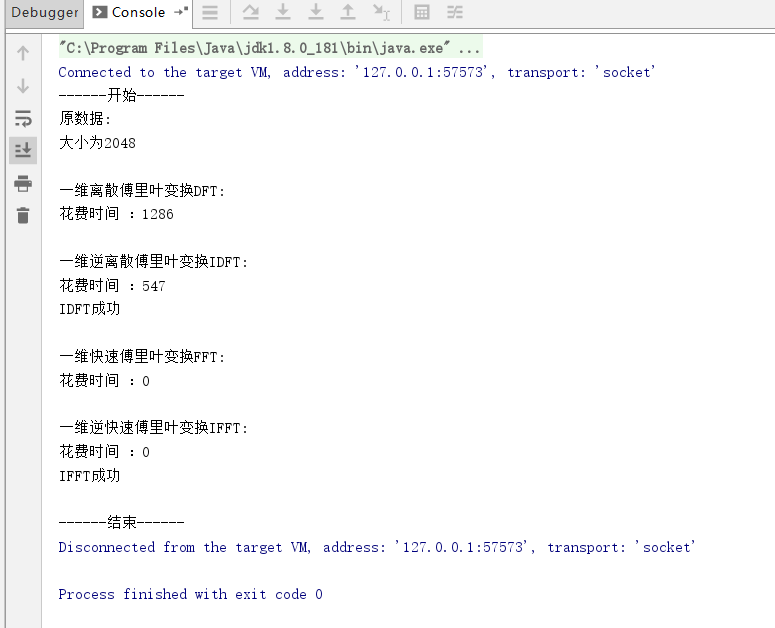4、将大小设为1百万，并将DFT和IDFT的代码注释掉。只要6s多。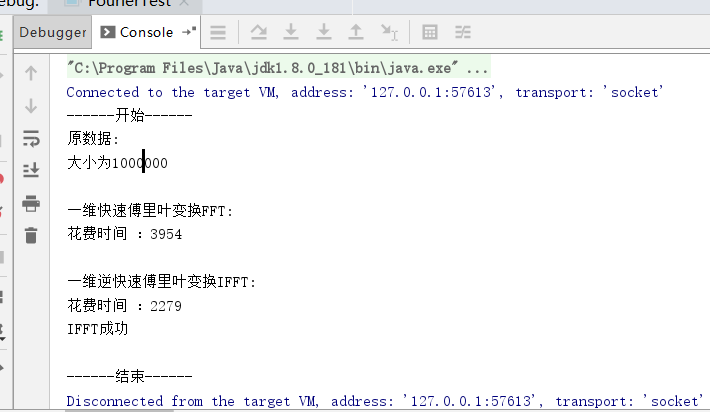展开全文FFT
• ## 傅里叶变换一维离散傅里叶变换

万次阅读 热门讨论 2019-11-06 21:08:43
DFT:(Discrete Fourier Transform)离散傅里叶变换是傅里叶变换在时域和频域上都呈离散的形式，将信号的时域采样变换为其DTFT的频域采样。在形式上，变换两端（时域和频域上）的序列是有限长的，而实际上这两组序列...
1、介绍。

DFT:(Discrete Fourier Transform)离散傅里叶变换是傅里叶变换在时域和频域上都呈离散的形式，将信号的时域采样变换为其DTFT的频域采样。在形式上，变换两端（时域和频域上）的序列是有限长的，而实际上这两组序列都应当被认为是离散周期信号的主值序列。即使对有限长的离散信号作DFT，也应当将其看作其周期延拓的变换。实际应用的时候，都是使用快速傅里叶变换的，因为运算速度快。

1）、欧拉公式：

，其中i是虚数，即i的平方为-1。

2）、一维离散傅里叶变换DFT公式：

u是转换后一维数组的位置，F(u)是转换后数组中相应位置的值。x是原一维数组的位置，f(x)是原数组中相应的值。

，其中i是虚数。

一维DFT公式中的就是欧拉公式中的，而且，。

所以一维DFT公式又可以写成：。

3）、一维离散傅里叶逆变换IDFT公式：

x是转换后一维数组的位置，f(x)是转换后数组中相应位置的值。u是原一维数组的位置，F(u)是原数组中相应的值。

。

又可以写成：。

2、生成随机的一维数组。

    //f(x)=1+2x
private static double[] getRandom(int length) {
double[] array = new double[length];
for (int x = 0; x < length; x++) {
double value = 1 + 2 * x;
array[x] = value;
}
return array;
}

3、复数的类。参考文章高数 复数的四则运算

package com.zxj.reptile.utils.number;

public class Complex {
private double real;//实数
private double image;//虚数

public Complex() {
real = 0;
image = 0;
}

public Complex(double real, double image) {
this.real = real;
this.image = image;
}

//加：(a+bi)+(c+di)=(a+c)+(b+d)i
double real = complex.getReal();
double image = complex.getImage();
double newReal = this.real + real;
double newImage = this.image + image;
return new Complex(newReal, newImage);
}

//减：(a+bi)-(c+di)=(a-c)+(b-d)i
public Complex sub(Complex complex) {
double real = complex.getReal();
double image = complex.getImage();
double newReal = this.real - real;
double newImage = this.image - image;
return new Complex(newReal, newImage);
}

public Complex mul(Complex complex) {
double real = complex.getReal();
double image = complex.getImage();
double newReal = this.real * real - this.image * image;
double newImage = this.image * real + this.real * image;
return new Complex(newReal, newImage);
}

public Complex mul(double multiplier) {
return mul(new Complex(multiplier, 0));
}

public Complex div(Complex complex) {
double real = complex.getReal();
double image = complex.getImage();
double denominator = real * real + image * image;
double newReal = (this.real * real + this.image * image) / denominator;
double newImage = (this.image * real - this.real * image) / denominator;
return new Complex(newReal, newImage);
}

//欧拉公式 e^(ix)=cosx+isinx
public static Complex euler(double x) {
double newReal = Math.cos(x);
double newImage = Math.sin(x);
return new Complex(newReal, newImage);
}

public double getReal() {
return real;
}

public void setReal(double real) {
this.real = real;
}

public double getImage() {
return image;
}

public void setImage(double image) {
this.image = image;
}

@Override
public String toString() {
String str = "";
if (real != 0) {
str += real;
} else {
str += "0";
}
if (image < 0) {
str += image + "i";
} else if (image > 0) {
str += "+" + image + "i";
}
return str;
}
}

4、一维离散傅里叶变换DFT和一维逆离散傅里叶变换IDFT代码。

    /**
* 一维离散傅里叶变换DFT
*
* @param array 一维数组
*/
public static Complex[] getDft(double[] array) {
Complex[] complexArray = Complex.getComplexArray(array);
return dftProgress(complexArray, -1);
}

/**
* 一维逆离散傅里叶变换IDFT
*
* @param complexArray 一维复数数组
*/
public static double[] getInverseDft(Complex[] complexArray) {
int length = complexArray.length;
Complex[] resultArray = dftProgress(complexArray, 1);
double[] array = new double[length];
for (int i = 0; i < length; i++) {
array[i] = NumberUtils.getRound(resultArray[i].getReal() / length, 2);
}
return array;
}

/**
* 一维离散傅里叶变换DFT和一维逆离散傅里叶变换IDFT计算过程
*
* @param array 复数数组
* @param minus 正负值，DFT=-1，IDFT=1
*/
private static Complex[] dftProgress(Complex[] array, int minus) {
int length = array.length;
Complex[] complexArray = new Complex[length];
// minus * 2 * PI / N
double flag = minus * 2 * Math.PI / length;
for (int i = 0; i < length; i++) {
Complex sum = new Complex();
for (int j = 0; j < length; j++) {
//array[x] * e^((minus * 2 * PI * k / N)i)
Complex complex = Complex.euler(flag * i * j).mul(array[j]);
}
//累加
complexArray[i] = sum;
}
return complexArray;
}

5、流程。可以先将随机数组的大小设小点，然后将打印日志的代码开起来，这样子才会对流程有更多的了解。

    public static void main(String[] args) {
System.out.println("------开始------");
long time = System.currentTimeMillis();
testDft(3000);//一维离散傅里叶变换
System.out.println("花费时间 ：" + (System.currentTimeMillis() - time));
System.out.println("------结束------");
}

private static void testDft(int length) {
//System.out.println("原数据: ");
double[] array = getRandom(length);
for (int i = 0; i < array.length; i++) {
//System.out.println(array[i]);
}
//System.out.println("离散傅里叶变换DFT: ");
Complex[] dtfArray = FourierUtils.getDft(array);
for (int i = 0; i < dtfArray.length; i++) {
//System.out.println(dtfArray[i]);
}
//System.out.println("逆离散傅里叶变换IDFT: ");
double[] inverseDtfArray = FourierUtils.getInverseDft(dtfArray);
for (int i = 0; i < inverseDtfArray.length; i++) {
//System.out.println(inverseDtfArray[i]);
}
System.out.print("一维离散傅里叶变换和逆离散傅里叶变换");
if (Arrays.equals(array, inverseDtfArray)) {
System.out.println("成功");
} else {
System.out.println("失败");
}
}

6、结果。可以将生成随机一维数组的大小进行改变，也可以对生成随机数据的函数进行改变。会发现原数组和经过离散傅里叶变换DFT及逆离散傅里叶变换IDFT之后的数组是一模一样的，就可以验证出算法和公式是对的。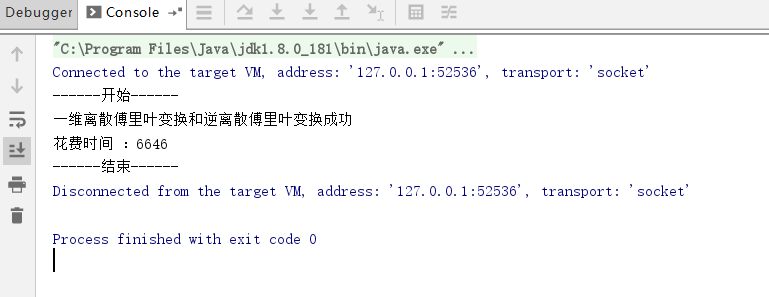展开全文IDFT
• ## 傅里叶变换(二维离散傅里叶变换)

万次阅读 多人点赞 2018-06-15 22:22:35
（1）可分离性： 二维离散傅里叶变换DFT可分离性的基本思想是DFT可分离为两次一维DFT。因此可以用通过计算两次一维的FFT来得到二维快速傅里叶FFT算法。根据快速傅里叶变换计算要求，需要图像的行数、列数均满足2...
离散二维傅里叶变换一常用性质：       可分离性、周期性和共轭对称性、平移性、旋转性质、卷积与相关定理；（1）可分离性：   二维离散傅里叶变换DFT可分离性的基本思想是DFT可分离为两次一维DFT。因此可以用通过计算两次一维的FFT来得到二维快速傅里叶FFT算法。根据快速傅里叶变换的计算要求，需要图像的行数、列数均满足2的n次方，如果不满足，在计算FFT之前先要对图像补零以满足2的n次。   一个M行N列的二维图像f(x,y)，先按行队列变量y做一次长度为N的一维离散傅里叶变换，再将计算结果按列向对变量x做一次长度为M傅里叶变换就可以得到该图像的傅里叶变换结果，如式所示：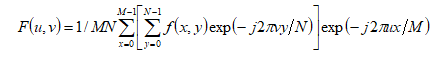将上式分解开来就是如下的两部分，先得到F(x,v),再由F(x,v)得到F(u,v)：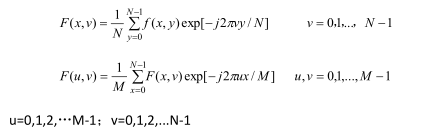计算过程如下：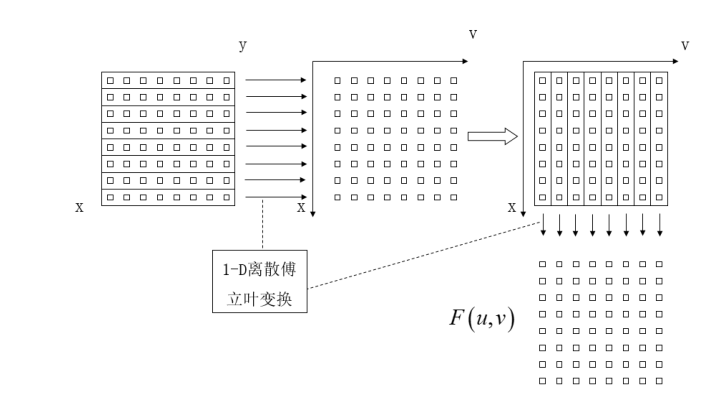每一行由N个点，对每一行的一维N点序列进行离散傅里叶变换得到F(x,u),再对得到F(x,u)按列向对每一列做M点的离散傅里叶变换，就可以得到二维图像f(x,y)的离散傅里叶变换F(u,v).同样，做傅里叶逆变换时，先对列向做一维傅里叶逆变换，再对行做一维逆傅里叶变换，如下式所示：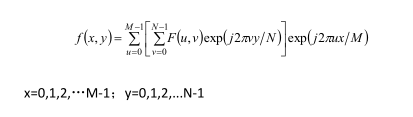（2）周期性和共轭对称性由傅里叶变换的基本性质可以知道，离散信号的频谱具有周期性。离散傅里叶变换DFT和它的里变换都以傅里叶变换的点数N为周期的。对于一维傅里叶变换有：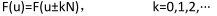对于二维傅里叶变换有：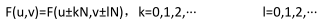类似有：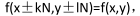即从DFT角度来看，反变换得到的图像阵列也是二维循环的。共轭对称性对于一维信号有：F(u)=F*(-u),如图所示的一维信号的幅度谱：点数为M的傅里叶变换一个周期为M，关于原点对称。原点即为0频率点，从图中可以看出在0频率的值最大，即信号f(x)的直流分量(均值)，远离原点处的即为高频成份，高频成份的幅值较小，说明信号的大部分能量集中在低频部分。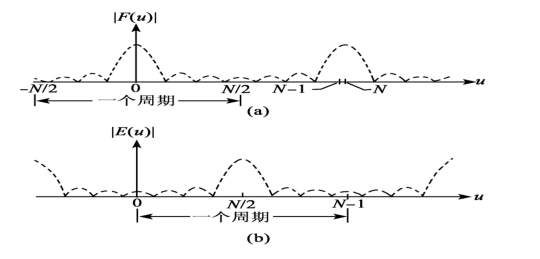对于二维信号有：F(u,v)=F*(-u,-v)对于二维图像，其结果如图c所示。左上角(0,0)处为二维图像得0频率点，该点得值对应图像的平均灰度值，图中四个角对应低频成分，中间区域为高频成份，低频区域的幅度值打羽高频区域的幅度值，也同样表示该信号的主要能量集中在低频区域。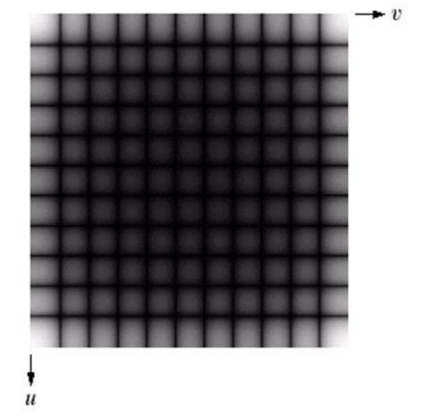根据周期性和共轭对称性，在对图像进行频谱分析处理时只需要关注一个周期就可以了，同时利用图像的傅里叶变换和傅里叶变换的共轭可以直接计算图像的幅度谱，因此使得图像的频谱计算和显示得以简化。（3)平移性：傅里叶变换对有如下平移性质：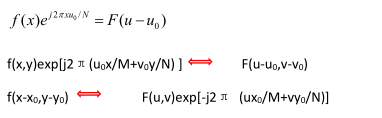式子表明，在频域中原点平移到(u0 ,v0)时，其对应的空间域 f(x,y)要乘上一个正的指数项：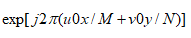在空域中图像原点平移到(x0,y0)时，其对应的F(u,v)要乘上一个负的指数项：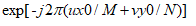在数字图像处理中，常常需要将F(u,v)的原点移到N*N频域的中心，以便能清楚地分析傅里叶谱的情况，平移前空域、频域原点均在左上方。要做到这点，只需令上面平移公式中的：u0=v0=N/2；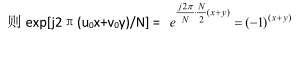所以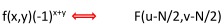上式表明：如果需要将图像傅里叶谱的原点从左上角(0,0)移到中心点(N/2,N/2),只要f(x,y)乘上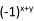因子进行傅里叶变换即可实现。平移性还体现了：当空域中f(x,y)产生移动时，在频域中只发生相移，并不影响他的傅里叶变换的幅度，因为：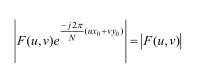反之，当频域中F(u,v)产生移动时，相应f(x,y)在空域中也只发生相移，不产生幅值变化。根据平移性质，为了更清楚查看二维图像的频谱，使直流成分出项在图像中央，在把画面分成四分的基础上，进行如图所示的换位(移位)也是可以的，这样，频域原点就回平移到中心。如下所示：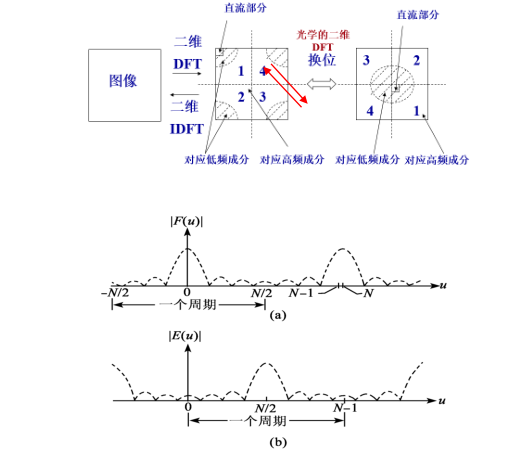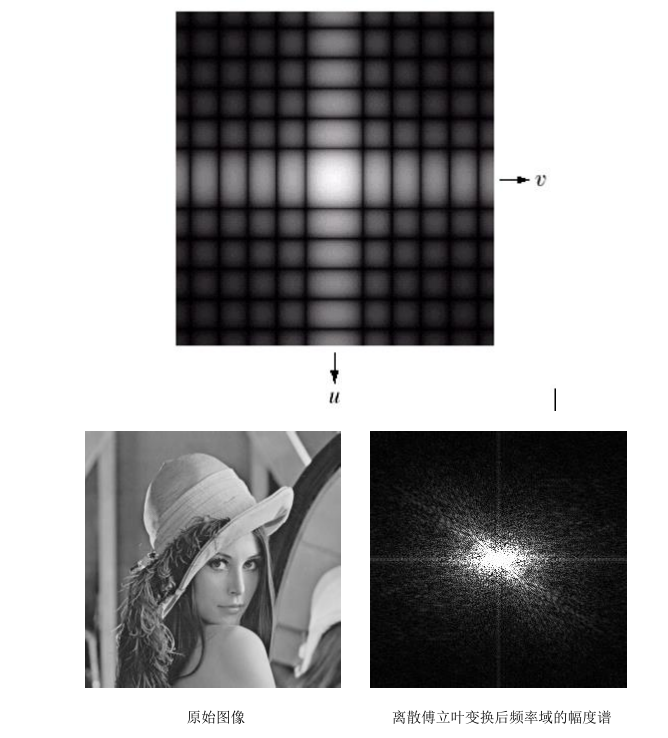（4）旋转性质如果 f(x,y)旋转了一个角度，那么 f(x,y)旋转后的图像的傅立叶变换也旋转了相同的角度。平面直角坐标改写成极坐标形式：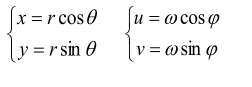替换则有：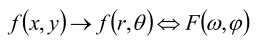如果f(x,y)被旋转W,则F(u,v)被旋转同一角度。即有傅里叶变换对：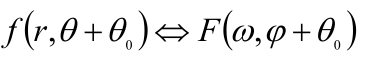如下所示：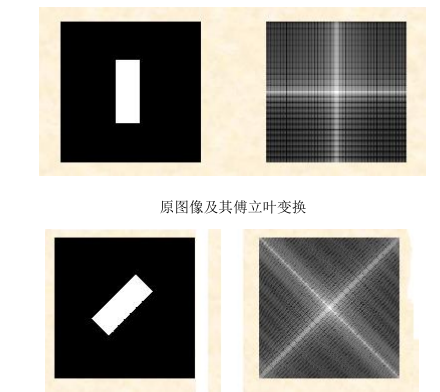同时，我们可以得出结论，对图像进行旋转变换和傅立叶变换的顺序是可交换的。即先旋转再傅里叶变换或者先傅里叶变换再旋转，得到的结果相同。F{R{f(x,y)}} = R{F{f(x,y)}}。（5）卷积与相关定理卷积定理包括空间域卷积和频率域卷积，卷积是空间域滤波和频率域滤波之间的纽带：两个空域信号的卷积等价于其频域信号的 乘积f(x,y)*h(x,y) → F(u,v)H(u,v) 或者 F{f(x,y)*h(x,y)} = F(u,v)H(u,v)；两个信号频域上的卷积等价于空间域的相乘f(x,y) g(x,y) →F(u,v)*H(u,v)；该性质的好处是将需要经过翻折、平移、相乘、求和等步骤实现的复杂的卷积运算简化为简单的乘法运算，这也是快速傅里叶变换（FFT）的出现使得该性质得到更广泛应用，同时，该性质对于理解信号的频率域处理方法特别重要，使得信号的空间域处理可以转换到频率域进行处理实现。根据空间域卷积定理，在空间域对应的是原始信号与滤波器的冲击响应的卷积，卷积定义式为信号翻折平移求和的过程，步骤复杂，运算量大，但如果转换到频率域进行处理，则对在将二者的频谱直接相乘就可以得到滤波结果，然后对滤波结果进行傅里叶逆变换就可以得到滤波后的空间域域图像。如下图所示，对信号进行低通和高通滤波处理的过程和效果。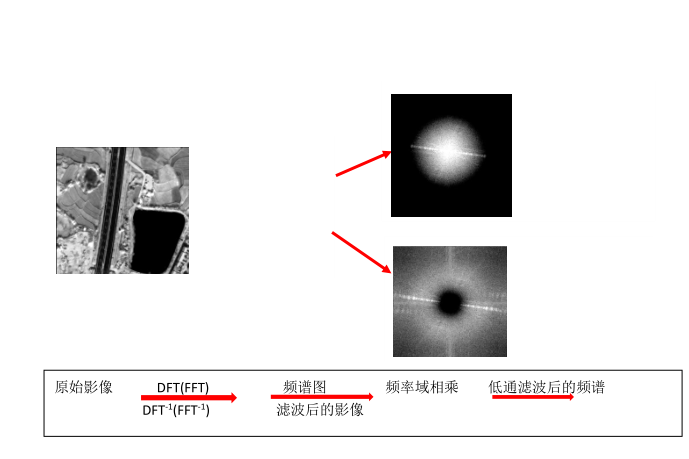相关定理：空域中 f(x,y)与 与 g(x,y) 的相关等价于频域中 F(u,v) 的共轭与 G(u,v)  相乘f(x,y) g(x,y) → F*(u,v)G(u,v)同时有：f*(x,y)g(x,y) → F(u,v) G(u,v)相关定理与卷积定理类似，也是把积分求和过程转化为了频域相乘，因此，也使得相关分析的计算简化。相关的重要应用在于匹配：确定是否有感兴趣的物体区域。f(x,y)是原始图像，g(x,y)作为感兴趣的物体或区域（模板），如果匹配，两个函数的相关值会在 f 中找到相应 g 点的位置上达到最大值。如下图所示。图像 f(x,y) 与模板 g(x,y)，通过计算相关函数，在匹配点处达到最大值，如图中红色圆圈标注的区域。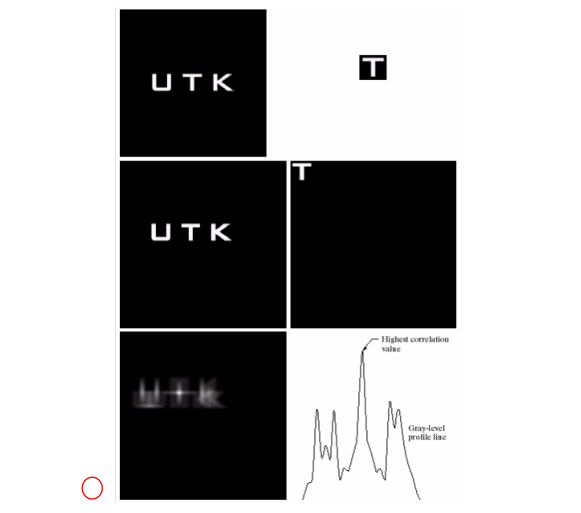延拓图像 f(x,y)，延拓图像 g(x,y)，相关函数图像，通过相关图像最大值的水平灰度剖面图。傅里叶变换的实例与应用首先我们认识几点有关傅里叶变换的特点：l 傅里叶变换是从将图像从空间域变换到频率域，具有明确的物理意义。图像的频率是表征图像中灰度变化剧烈程度的指标，是灰度在平面空间上的梯度，在噪声点和图像边缘处的频率为高频。l 在频率域中，将信号表示为一系列正弦信号或者复指数函数的叠加，正弦信号的频率、幅值和相位可以描述正弦信号中的所有信息，由此可以得到信号的幅度谱和相位谱。在图像领域就是将图像灰度作为正弦变量。l 傅里叶变换全局性的，是一个积分求和的过程，对时间、地点位置无法进行准确定义，也就是说傅里叶变换得到的频谱图中的点无法与空间域中的某个空间位置对应，因此，从傅里叶变换图中并不能直接对应某个位置的特点。l 傅里叶变换是一系列不同频率三角函数的和，每个频率分量的系数不同，这些系数代表了各频率成分的强弱或者所占比重，通过分析这些系数就可以分析图像的特性。
展开全文• ## 一维离散傅里叶变换，信号分解

万次阅读 热门讨论 2018-04-03 11:38:53
如下例子处理一维离散信号信号分析通过傅里叶变换，可以将实变信号f(t)分解成各个频率分量的线性叠加，进而从频率的角度研究信号的组成。来看这个杂乱无章的曲线图，你是否能看出它的规律？Figure 1 What's ...
 傅里叶变换的应用涵盖了概率与统计、信号处理、量子力学和图像处理等学科。离散傅里叶变换的公式如下：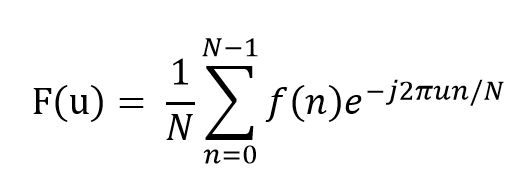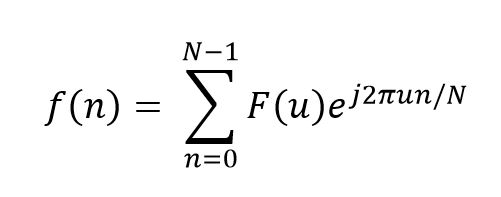在MATLAB中，可以直接使用函数库fft(X)对一维向量X做傅里叶变换，分析信号的组成。如下例子处理一维离散信号信号分析通过傅里叶变换，可以将实变信号f(t)分解成各个频率分量的线性叠加，进而从频率的角度研究信号的组成。来看这个杂乱无章的曲线图，你是否能看出它的规律？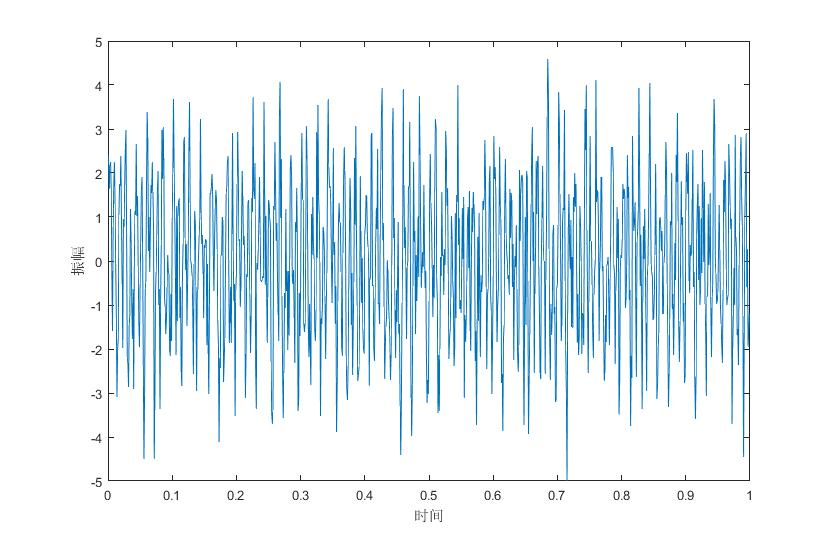Figure 1 What's the law of the signal?让我们来对它做一维离散傅里叶变换，得到下图：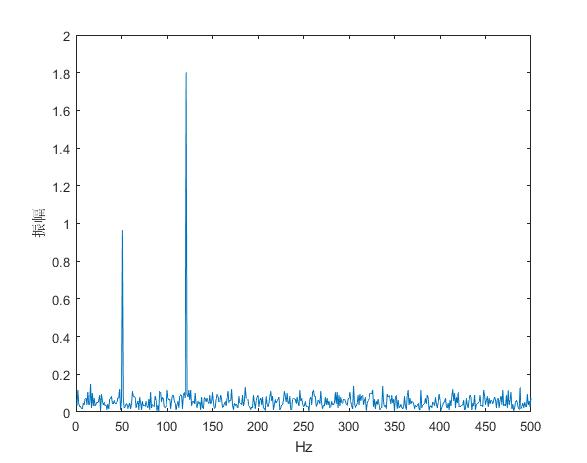Figure 2 The Fourier Transform of the signal in the figure 1从图2的傅里叶变换中我们可以观察到信号在频率为50Hz和120Hz的地方有峰值，表明了原信号主要由频率为50Hz和120Hz的三角函数信号组成。上面例子的具体MATLAB代码如下：clear
Fs = 1000;            % Sampling frequency
T = 1/Fs;             % Sampling period
L = 1000;             % Length of signal
t = (0:L-1)*T;        % Time vector

S = 0.9*sin(2*pi*50*t) + 1.8*sin(2*pi*120*t); %standard signal
X = S + 1*randn(size(t)); %there are some noise mixing in the signal.

Y = fft(X);           % Fourier Transform of the signal

f = 1:L/2;            % the frequence of label
% half of Y is enough to show the information.
Y = Y./L*2;           % calculate the amplitude of Fourier Transform of the signal

%draw the curve
figure(1);
plot(t,X);            % show the original signal
xlabel('时间')
ylabel('振幅')
figure(2);
plot(f,abs(Y(1:L/2)));% show the Fourier Transform of the signal
xlabel('Hz');
ylabel('振幅');傅里叶变换可以把信号的振动频率分离出来，我们可以通过设置不同频率分量前的系数，可实现信号的滤波。如含有噪声的信号：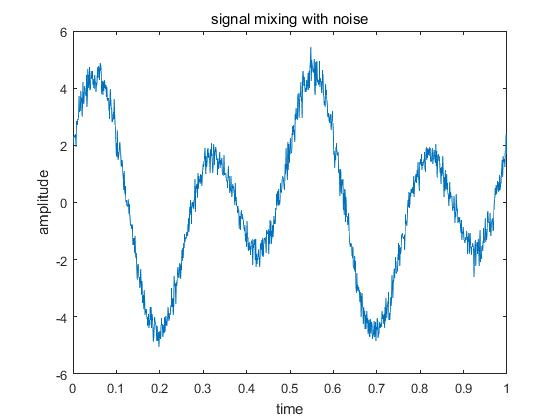Figure 3 signal mixing with noise将该信号进行傅里叶变换，然后使得高频信号的系数为零，再傅里叶反变换回原信号，可得到滤波后的结果：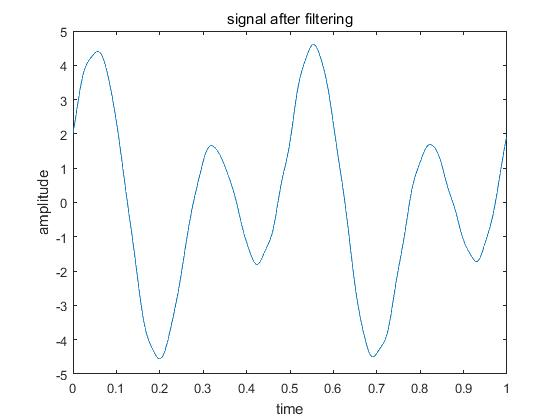Figure 4 signal after filtering要注意的是，要想使用好傅里叶变换，必须对傅里叶变换有较为深入的了解，比如上面的滤波中，如果设置滤波频率的界限不同，那么其滤波效果也不同；还要注意的是，傅里叶变换后的信号具有周期性，其数据关于中心位置对称，所以滤波界限要关于中心对称；上例中具体实现代码如下：clear
Fs = 1000;
T = 1./Fs;
L = 1000;
t = (0:L-1)*T;
S = 2*cos(2*pi*2*t)+3*sin(2*pi*4*t);    %standard signal without the noise
X = S+0.3*randn(size(t));               %signal mixing with noise
figure(1);
plot(t,X);
title('signal mixing with noise');
xlabel('time');
ylabel('amplitude');

Y1 = fft(X);                            %fourier transform

X1 = ifft(Y1);                          %Inverse Fourier transform
figure(2);
plot(t,X1);
title('signal after filtering');
xlabel('time');
ylabel('amplitude');本主要讲了离散傅里叶变换的两个应用——分析信号组成和滤波，其用处还很广泛，比如数据压缩、求微分方程等等。
展开全文MATLAB 滤波
• 如下例子处理一维离散信号信号分析通过傅里叶变换，可以将实变信号f(t)分解成各个频率分量的线性叠加，进而从频率的角度研究信号的组成。来看这个杂乱无章的曲线图，你是否能看出它的规律？ Figure 1What'...
• 本文介绍如何用C++实现一维离散傅里叶变换（Discrete Fourier Transform）。 1. 一维傅里叶变换公式简介 如果读者对傅里叶变换的认识处于一知半解的程度，那么建议先阅读相关文章，例如网络上广泛流传的《如果看了...图像处理 算法
• . 欧拉公式 二. 傅里叶变换 N为采样的总个数，也就是样本的总个数 应用欧拉公式 三. 傅里叶变换 公式 四. c++编程实现 #include<iostream> #include<cmath> #include<iomanip> using...算法
• ## 傅里叶变换 二维离散傅里叶变换

万次阅读 热门讨论 2019-11-07 15:41:28
DFT:(Discrete Fourier Transform)离散傅里叶变换是傅里叶变换在时域和频域上都呈离散的形式，将信号的时域采样变换为其DTFT的频域采样。在形式上，变换两端（时域和频域上）的序列是有限长的，而实际上这两组序列...二维DFT
• 第一章：一维离散卷积假设有两个限序列：，我们把他们放入以下栅格：然后，沿着，进行滑窗： 从下面开始出现相互重叠的栅格： 直到不再有相互重叠的栅格：矩阵法求卷积：首先给出矩阵构造的方法步骤：然后在末尾...一维离散卷积 opencv 卷积定理
• 文章目录一维离散傅里叶变换二维离散傅里叶变换一维快速傅里叶变换二维FFTc语言代码结果 一维离散傅里叶变换 F(u)=∑x=0M−1f(x)e−j2πux/M,u=0,1,2,...M−1F(u)=\sum_{x=0}^{M-1}f(x)e^{-j2\pi ux/M} ,u=0,1,2,......
• 维离散傅里叶变换DFT matlab代码图像处理领域离散傅里叶变换的作用二维离散傅里叶变换维离散傅里叶变换公式将二维的离散傅里叶变换进行转化将系数转化为矩阵形式注意，从矩阵的乘积i形式可以看出，原来是N个值，...图像处理代码 matlab
• 从一维连续Fouier变换和逆变换公式推导一维离散Fourier变换和逆变换公式，并对得到的公式进行系数修正。
• 维离散傅里叶变换3.1 二维离散傅里叶变换(DFT) 3.1.1 二维连续傅里叶变换 二维连续函数 f (x, y)的傅里叶变换定义如下： 设 是独立变量 的函数，且在 上绝对可积，则定义积分 为二维连续函数 的付里叶变换，并定义...
• 应用：二维离散傅里叶变换是将图像从空间域转至频域，在图像增强、图像去噪、图像边缘检测、图像特征提取、图像压缩等等应用中都起着极其重要的作用。 **理论基础：**任意函数都可以表示成正弦函数的线性组合的形式...
• 1、一维FFT和二维FFT。 一维DFT公式： 。 二维DFT公式： 。 可以看作M行N列的二维数组，先对每行做一维FFT，将结果作为一个新的二维数组。再对新的二维数组每列做一维FFT。而在处理二维IFFT的时候，跟二维...FFT
• 在学完一维傅里叶变换后，紧接着就是二维的傅里叶变换了。直接上干货吧！！！ 途中会用到opencv读取与显示图片。 一. 公式 M表示图像的行数，N表示图像的列数。 经过欧拉公式可以得一下形式，这样就可以轻松得到...C++
• 计算以下图像的离散傅里叶变换 f= f=\begin{bmatrix}1&4&4&1\\2&4&4&2\\2&4&4&2\\1&4&4&1\end{bmatrix} f=⎣⎢⎢⎡​1221​4444​4444​1221​...
• 应用：二维离散傅里叶变换是将图像从空间域转至频域，在图像增强、图像去噪、图像边缘检测、图像特征提取、图像压缩等等应用中都起着极其重要的作用。理论基础：任意函数都可以表示成正弦函数的线性组合的形式。二...
• 在上一篇文章《C++实现一维离散傅里叶变换》中，我们介绍了一维信号傅立叶变换的公式和C++实现，并阐述了频域幅值的意义。 一维傅立叶变换只适用于一维信号，例如音频数据、心脑电图等。 在图像处理中，图像信号...
• 二维傅里叶变换 我们先来看看一维情况的傅里叶变换。在信号系统中讲过连续时间的傅里叶变换和离散时间的傅里叶变换，连续时间傅里叶变换在频谱上时非周期的，离散时间傅里叶变换...我们其实可以将二维离散傅里叶...数字图像处理 循环卷积
• 1、一维DFT和二维DFT。 一维DFT公式： 。 二维DFT公式： 。 可以看作M行N列的二维数组，先对每行做一维DFT，将结果作为一个新的二维数组。再对新的二维数组每列做一维DFT。 而在处理二维IDFT的时候，跟二维...二维DFT
• 实际上对图像进行二维傅里叶变换得到频谱图，就是图像梯度的分布图，傅里叶频谱图上看到的明暗不一的亮点就是某一点与领域差异的强弱，即梯度的大小（该点的频率大小）。 .原理 1、代数形式的傅里叶变换： 2...
• 想起来我还写了个计算维离散傅里叶变换的代码 要用opencv #include "opencv2/core.hpp" #include "opencv2/core/utility.hpp" #include "opencv2/imgproc.hpp" #include "opencv2/highgui.hpp" #include<......